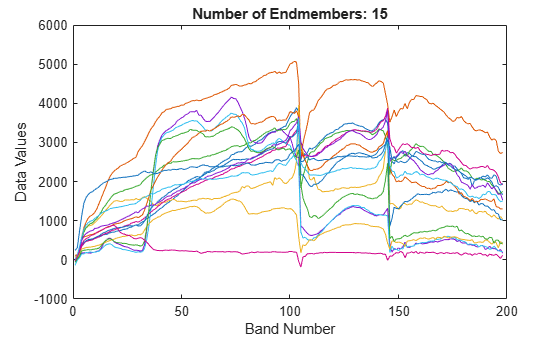# countEndmembersHFC

Find number of endmembers

Since R2020a

## Syntax

``numEndmembers = countEndmembersHFC(inputData)``
``numEndmembers = countEndmembersHFC(inputData,Name,Value)``

## Description

example

````numEndmembers = countEndmembersHFC(inputData)` finds the number of endmembers present in a hyperspectral data cube by using the noise-whitened Harsanyi–Farrand–Chang (NWHFC) method.```

example

````numEndmembers = countEndmembersHFC(inputData,Name,Value)` specifies additional options using one or more name-value pair arguments. For example, `'NoiseWhiten',false` does not perform noise-whitening of the data before extracting the endmembers. NoteThis function requires the Image Processing Toolbox™ Hyperspectral Imaging Library. You can install the Image Processing Toolbox Hyperspectral Imaging Library from Add-On Explorer. For more information about installing add-ons, see Get and Manage Add-Ons.The Image Processing Toolbox Hyperspectral Imaging Library requires desktop MATLAB®, as MATLAB Online™ or MATLAB Mobile™ do not support the library. ```

## Examples

collapse all

Read hyperspectral data into the workspace.

`hcube = hypercube('jasperRidge2_R198.hdr');`

Find the number of endmembers in the hyperspectral data by using the NWHFC method.

`numEndmembers = countEndmembersHFC(hcube);`

Estimate the endmember spectra using the N-FINDR method.

`endmembers = nfindr(hcube,numEndmembers);`

Plot the endmember spectra.

```figure plot(endmembers) title(['Number of Endmembers: ' num2str(numEndmembers)]) xlabel('Band Number') ylabel('Data Values') ```Read hyperspectral data into the workspace.

`hcube = hypercube('jasperRidge2_R198.hdr');`

Find the number of endmembers in the hyperspectral data by using the HFC method. To use the HFC method, set the `'NoiseWhiten'` parameter value to `false`.

`numEndmembers = countEndmembersHFC(hcube,'NoiseWhiten',false);`

Estimate the endmember spectra using the N-FINDR method.

`endmembers = nfindr(hcube,numEndmembers);`

Plot the endmember spectra.

```figure plot(endmembers) title(['Number of Endmembers: ' num2str(numEndmembers)]) xlabel('Band Number') ylabel('Data Values') ```## Input Arguments

collapse all

Input hyperspectral data, specified as a 3-D numeric array that represent the hyperspectral data cube of size M-by-N-by-C or `hypercube` object. If the input is a `hypercube` object, the function reads the data cube stored in the `DataCube` property of the object. The hyperspectral data cube must be real and non-sparse.

Data Types: `single` | `double` | `int8` | `int16` | `int32` | `int64` | `uint8` | `uint16` | `uint32` | `uint64`

### Name-Value Arguments

Specify optional pairs of arguments as `Name1=Value1,...,NameN=ValueN`, where `Name` is the argument name and `Value` is the corresponding value. Name-value arguments must appear after other arguments, but the order of the pairs does not matter.

Before R2021a, use commas to separate each name and value, and enclose `Name` in quotes.

Example: `countEndmembersHFC(inputData,'NoiseWhiten',false)`

Probability of false alarm, specified as the comma-separated pair consisting of `'PFA'` and a positive scalar in the range (0, 1].

Data Types: `single` | `double` | `int8` | `int16` | `int32` | `int64` | `uint8` | `uint16` | `uint32` | `uint64`

Perform noise-whitening, specified as the comma-separated pair consisting of `'NoiseWhiten'` and a numeric or logical `1` (`true`) or `0` (`false`).

• `true` or `1` — Perform noise-whitening of input data before computing the number of endmembers. This approach is the NWHFC method.

• `false` or `0` — Do not perform noise-whitening of input data before computing the number of endmembers. This approach is the Harsanyi–Farrand–Chang (HFC) method.

Data Types: `single` | `double` | `int8` | `int16` | `int32` | `int64` | `uint8` | `uint16` | `uint32` | `uint64` | `logical`

## Output Arguments

collapse all

Number of endmembers in the hyperspectral data, returned as a positive numeric scalar.

Data Types: `double`

 Chang, C.-I., and Q. Du. “Estimation of Number of Spectrally Distinct Signal Sources in Hyperspectral Imagery.” IEEE Transactions on Geoscience and Remote Sensing 42, no. 3 (March 2004): 608–19. https://doi.org/10.1109/TGRS.2003.819189.